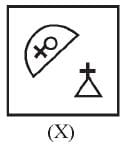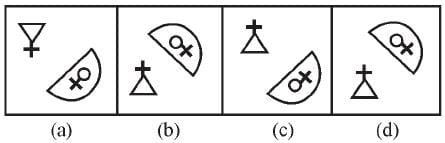Olympiad Test : Mirror Images - 1

# Olympiad Test : Mirror Images - 1

Test Description

## 20 Questions MCQ Test Maths Olympiad Class 6 | Olympiad Test : Mirror Images - 1

Olympiad Test : Mirror Images - 1 for Class 6 2023 is part of Maths Olympiad Class 6 preparation. The Olympiad Test : Mirror Images - 1 questions and answers have been prepared according to the Class 6 exam syllabus.The Olympiad Test : Mirror Images - 1 MCQs are made for Class 6 2023 Exam. Find important definitions, questions, notes, meanings, examples, exercises, MCQs and online tests for Olympiad Test : Mirror Images - 1 below.
 1 Crore+ students have signed up on EduRev. Have you?
Olympiad Test : Mirror Images - 1 - Question 1

### In each of the following questions, there is combination of alphabet or number followed by four alternatives. Choose the alternative which most clearly resembles the mirror image of the given combination. TERMINATE

Olympiad Test : Mirror Images - 1 - Question 2

### In each of the following questions, there is combination of alphabet or number followed by four alternatives. Choose the alternative which most clearly resembles the mirror image of the given combination. 1965INDOPAK

Olympiad Test : Mirror Images - 1 - Question 3

### In each of the following questions, there is combination of alphabet or number followed by four alternatives. Choose the alternative which most clearly resembles the mirror image of the given combination. NATIONAL

Olympiad Test : Mirror Images - 1 - Question 4

In each of the following questions, there is combination of alphabet or number followed by four alternatives. Choose the alternative which most clearly resembles the mirror image of the given combination.
UTZFY6KH

Olympiad Test : Mirror Images - 1 - Question 5

In each of the following questions, there is combination of alphabet or number followed by four alternatives. Choose the alternative which most clearly resembles the mirror image of the given combination.
SUPERVISOR

Olympiad Test : Mirror Images - 1 - Question 6

In each of the following questions, there is combination of alphabet or number followed by four alternatives. Choose the alternative which most clearly resembles the mirror image of the given combination.
JUDGEMENT

Olympiad Test : Mirror Images - 1 - Question 7

In each of the following questions, there is combination of alphabet or number followed by four alternatives. Choose the alternative which most clearly resembles the mirror image of the given combination.
ANS43Q12

Olympiad Test : Mirror Images - 1 - Question 8

In each of the following questions, there is combination of alphabet or number followed by four alternatives. Choose the alternative which most clearly resembles the mirror image of the given combination.
BRISK

Olympiad Test : Mirror Images - 1 - Question 9

In each of the following questions, there is combination of alphabet or number followed by four alternatives. Choose the alternative which most clearly resembles the mirror image of the given combination.
EFFECTIVE

Olympiad Test : Mirror Images - 1 - Question 10

In each of the following questions, there is combination of alphabet or number followed by four alternatives. Choose the alternative which most clearly resembles the mirror image of the given combination.
TARAIN1014A

Olympiad Test : Mirror Images - 1 - Question 11

In each of the following questions, there is combination of alphabet or number followed by four alternatives. Choose the alternative which most clearly resembles the mirror image of the given combination.
MAGAZINE

Olympiad Test : Mirror Images - 1 - Question 12

In each of the following questions, there is combination of alphabet or number followed by four alternatives. Choose the alternative which most clearly resembles the mirror image of the given combination.
BR4AQ16HI

Olympiad Test : Mirror Images - 1 - Question 13

In each of the following questions, there is combination of alphabet or number followed by four alternatives. Choose the alternative which most clearly resembles the mirror image of the given combination.
DL9CG4728

Olympiad Test : Mirror Images - 1 - Question 14

In each of the following questions, there is combination of alphabet or number followed by four alternatives. Choose the alternative which most clearly resembles the mirror image of the given combination.
IMFORMATIONS

Olympiad Test : Mirror Images - 1 - Question 15

In each of the following questions, there is combination of alphabet or number followed by four alternatives. Choose the alternative which most clearly resembles the mirror image of the given combination.
REASONING

Olympiad Test : Mirror Images - 1 - Question 16

In each of the following questions, there is combination of alphabet or number followed by four alternatives. Choose the alternative which most clearly resembles the mirror image of the given combination.
AN54WMG3

Olympiad Test : Mirror Images - 1 - Question 17

In each of the following questions, choose the correct mirror image of the figure (X) from amongst the four alternatives (a), (b), (c) and (d) given along with Fig. (X).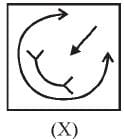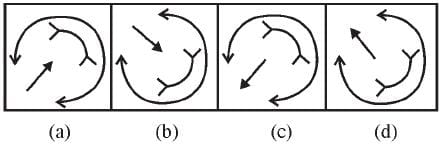Olympiad Test : Mirror Images - 1 - Question 18

In each of the following questions, choose the correct mirror image of the figure (X) from amongst the four alternatives (a), (b), (c) and (d) given along with Fig. (X).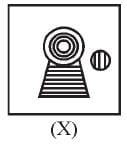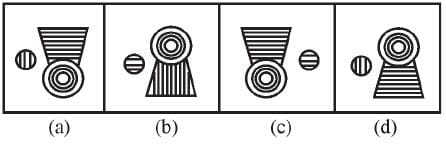Olympiad Test : Mirror Images - 1 - Question 19

In each of the following questions, choose the correct mirror image of the figure (X) from amongst the four alternatives (a), (b), (c) and (d) given along with Fig. (X).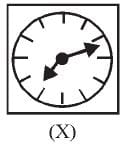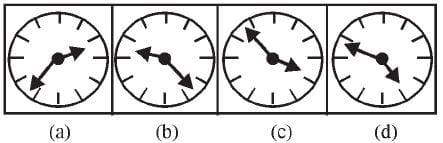Olympiad Test : Mirror Images - 1 - Question 20

In each of the following questions, choose the correct mirror image of the figure (X) from amongst the four alternatives (a), (b), (c) and (d) given along with Fig. (X).